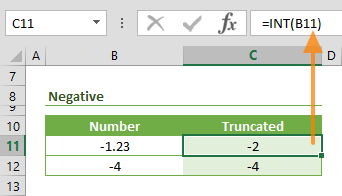The Excel INT function is a Math formula that rounds a given number down to the nearest integer. Although, it is similar to the TRUNC function, they work differently when working with negative numbers. In this guide, we’re going to show you how to use the INT function and also go over some tips and error handling methods.

## Supported versions

• All Excel versions

INT(number)

## Arguments

 number The number you want to round down to the nearest integer.

## Examples

### Positive Numbers

The INT function simply returns the integer part of a positive number. Enter a cell reference, formula, or a static value for the number argument.

=INT(1.234) returns 1### Negative Numbers

If you use a negative number with the INT function, the return value will be different than the integer part of the number. For example, the following formula returns -2 while its integer part is -1. This difference stems from the fact that the INT function rounds numbers down to nearest integer.

=INT(-1.23) returns -2## Summary and Tips

• To extract the decimal part of a number, you can use the following formula:
=1.23-INT(1.23)
• If you need the integer part of a negative number, use the TRUNC function instead. For example,
=TRUNC(-1.567) returns -1